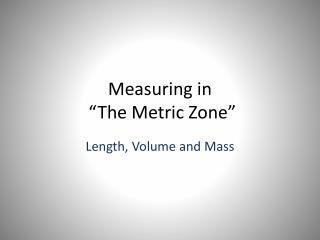# Measuring in “The Metric Zone” - PowerPoint PPT PresentationDownload PresentationMeasuring in “The Metric Zone”

Measuring in “The Metric Zone”
Download Presentation## Measuring in “The Metric Zone”

- - - - - - - - - - - - - - - - - - - - - - - - - - - E N D - - - - - - - - - - - - - - - - - - - - - - - - - - -
##### Presentation Transcript

1. Measuring in “The Metric Zone” Length, Volume and Mass

2. Length • Tools used in the lab most frequently: • Rulers • Meter sticks

3. A. 1 meter or 105 centimeters B. 4 kilometers or 4400 meters C. 12 centimeters or 102 millimeters D. 1200 millimeters or 1 meter km Metric Units m cm mm The basic unit of length in the metric system in the meter and is represented by a lowercase m. Standard: The distance traveled by light in absolute vacuum in 1⁄299,792,458 of a second. Metric Units 1 Kilometer (km) = 1000 meters 1 Meter = 100 Centimeters (cm) 1 Meter = 1000 Millimeters (mm) Which is larger?

4. 1 centimeter = 10 millimeters What is the length of the line in centimeters? _______cm What is the length of the line in millimeters? _______mm What is the length of the line to the nearest centimeter? ________cm HINT: Round to the nearest centimeter – no decimals. Measuring Length How many millimeters are in 1 centimeter? Ruler: http://www.k12math.com/math-concepts/measurement/ruler-cm.jpg

5. What causes the meniscus? A concave meniscus occurs when the molecules of the liquid attract those of the container. The glass attracts the water on the sides. Measuring Volume We will be using graduated cylinders to find the volume of liquids and other objects. Read the measurement based on the bottom of the meniscus or curve. When using a real cylinder, make sure you are eye-level with the level of the water. What is the volume of water in the cylinder? _____mL Top Image: http://www.tea.state.tx.us/student.assessment/resources/online/2006/grade8/science/images/20graphicaa.gifBottom Image: http://morrisonlabs.com/meniscus.htm

6. Measuring Liquid Volume What is the volume of water in each cylinder? Images created at http://www.standards.dfes.gov.uk/primaryframework/downloads/SWF/measuring_cylinder.swf A B C Pay attention to the scales for each cylinder.

7. kL Metric Units cL mL L Volume is the amount of space an object takes up. The base unit of volume in the metric system in the liter and is represented by L or l. We use milliliters ( mL) quite frequently in the lab. Metric Units 1 liter (L) = 1000 milliliters (mL) 1 milliliter (mL) = 1 cm3 (or cc) = 1 gram* Which is larger? A. 1 liter or 1500 milliliters B. 200 milliliters or 1.2 liters C. 12 cm3 or 1.2 milliliters* * When referring to waterLiter Image: http://www.dmturner.org/Teacher/Pictures/liter.gif

8. How do you measure Volume of a solid ? • To measure the volume of a solid. • Multiply : Length X Width X Height H E I G H T width length

9. Volume of an irregular shaped solid 1.0 mL = 1.0 cm3 51.0 mL 50.0 mL Water level Water level 1.0 cm3

10. 9 cm 8 cm 10 cm We can measure the volume of irregular object using water displacement. Amount of H2O with object = ______About of H2O without object = ______Difference = Volume = ______ http://resources.edb.gov.hk/~s1sci/R_S1Science/sp/en/syllabus/unit14/new/testingmain1.htm Measuring Solid Volume We can measure the volume of regular object using the formula length x width x height. _____ X _____ X _____ = _____

11. Kilogram Prototype A. 1 kilogram or 1500 grams B. 1200 milligrams or 1 gram C. 12 milligrams or 12 kilograms D. 4 kilograms or 4500 grams kg Metric Units cg mg g Mass refers to the amount of matter in an object. The base unit of mass in the metric system in the kilogram and is represented by kg. Standard: 1 kilogram is equal to the mass of the International Prototype Kilogram (IPK), a platinum-iridium cylinder kept by the BIPM at Sèvres, France. Metric Units 1 Kilogram (km) = 1000 Grams (g) 1 Gram (g) = 1000 Milligrams (mg) Which is larger? Kilogram Prototype Image - http://en.wikipedia.org/wiki/Kilogram

12. 1st – Place the film canister on the scale. 2nd – Slide the large weight to the right until the arm drops below the line. Move the rider back one groove. Make sure it “locks” into place. 3rd – Repeat this process with the top weight. When the arm moves below the line, back it up one groove. 4th – Slide the small weight on the front beam until the lines match up. Measuring Mass – Triple-Beam Balance 5th – Add the amounts on each beam to find the total mass to the nearest tenth of a gram.

13. Once you have balanced the scale, you add up the amounts on each beam to find the total mass. What would be the mass of the object measured in the picture? _______ + ______ + _______ = ________ g Measuring Mass We will be using triple-beam balances to find the mass of various objects. The objects are placed on the scale and then you move the weights on the beams until you get the lines on the right-side of the scale to match up. Top Image: http://www.southwestscales.com/Ohaus_Triple_Beam_750-SO.jpgBottom Image: http://www.regentsprep.org/Regents/biology/units/laboratory/graphics/triplebeambalance.jpg